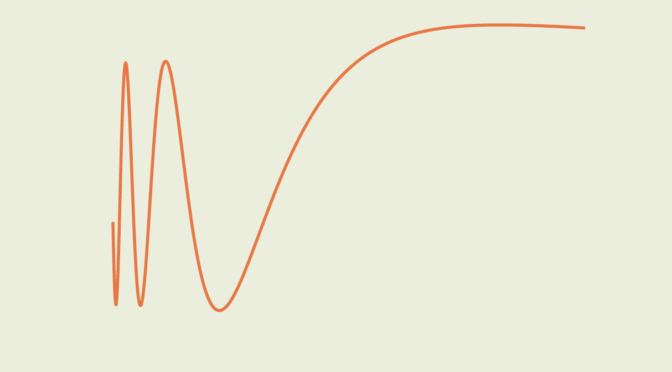# Converse of fundamental theorem of calculus

The fundamental theorem of calculus asserts that for a continuous real-valued function $$f$$ defined on a closed interval $$[a,b]$$, the function $$F$$ defined for all $$x \in [a,b]$$ by
$F(x)=\int _{a}^{x}\!f(t)\,dt$ is uniformly continuous on $$[a,b]$$, differentiable on the open interval $$(a,b)$$ and $F^\prime(x) = f(x)$
for all $$x \in (a,b)$$.

The converse of fundamental theorem of calculus is not true as we see below.

Consider the function defined on the interval $$[0,1]$$ by $f(x)= \begin{cases} 2x\sin(1/x) – \cos(1/x) & \text{ for } x \neq 0 \\ 0 & \text{ for } x = 0 \end{cases}$ $$f$$ is integrable as it is continuous on $$(0,1]$$ and bounded on $$[0,1]$$. Then $F(x)= \begin{cases} x^2 \sin \left( 1/x \right) & \text{ for } x \neq 0 \\ 0 & \text{ for } x = 0 \end{cases}$ $$F$$ is differentiable on $$[0,1]$$. It is clear for $$x \in (0,1]$$. $$F$$ is also differentiable at $$0$$ as for $$x \neq 0$$ we have $\left\vert \frac{F(x) – F(0)}{x-0} \right\vert = \left\vert \frac{F(x)}{x} \right\vert \le \left\vert x \right\vert.$ Consequently $$F^\prime(0) = 0$$.

However $$f$$ is not continuous at $$0$$ as it does not have a right limit at $$0$$.